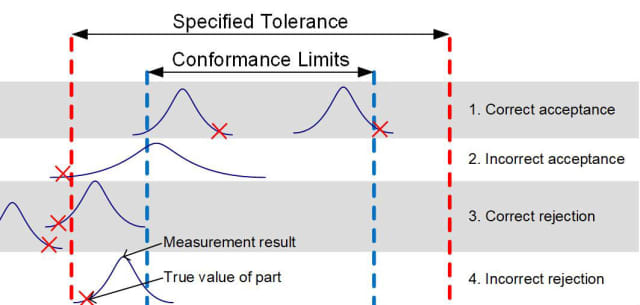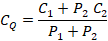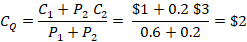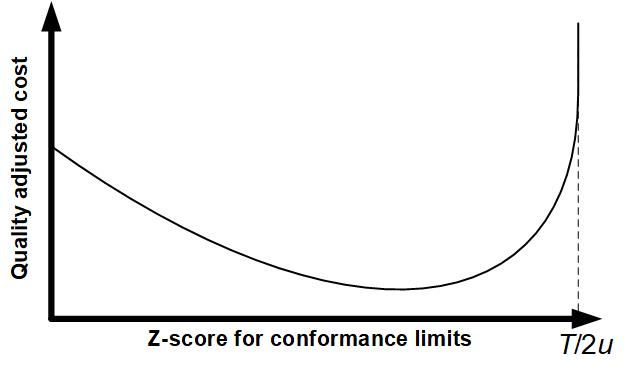Beyond Six-Sigma
Dr Jody Muelaner posted on February 28, 2019 |
What level of quality is most profitable?

Six-Sigma places a great deal of importance on the idea that processes must be ‘capable.’In this article, I’m going to turn that idea on its head. I believe that processes should be appropriate for the particular product being produced. In some cases that might mean you need to achieve one of the commonly recommended levels for process capability or a gage capability. For example, you might want a CPK of over 1.33 or a gage capability which is less than 30% of the product’s tolerance. In many cases, however, the most appropriate level of capability may be very different to the standard levels recommended within Six-Sigma and other standards, such as VDA-5. Most production engineers are well aware of this fact and often make judgements that a process is ‘good enough’ for a particular application even though it might not meet the recommended level of capability.

What’s missing from current quality engineering standards, including Six-Sigma, is a way to quantitatively evaluate what’s most appropriate for a particular application. Most of the time, what’s most appropriate is what’s most profitable. Therefore, when deciding on the most appropriate level of capability, it is important to consider how reducing quality might affect profit, as well as the cost of increasing capability.

Methods are now available which can weigh all of these considerations so that the production system can be configured to be as profitable as possible. This Cost-Optimized Quality approach uses optimization algorithms to select the most appropriate production process, measurement process, and rules for approving conformance.

### Considering the Process and the Gage Together

Conventionally, process capability and gage capability are looked at as two separate quantities. However, when a production process is very capable, a less capable measurement system may be acceptable, and vice versa. In the conventional approach to quality, the normal distribution is often used to determine how many defects we might expect for a given level of variation in the production process. When using a cost-optimized approach to quality, this can be taken a step further: using a bivariate normal distribution to determine the rate of different outcomes, considering both variation in the production process and uncertainty in the measurement system. When a part is produced, the true value of the part depends only on the variation of the production process, this may be described by a simple univariate normal distribution. The result of the measurement of this part, however, depends on both the true value of the part and the variation in the measurement system itself. Measurements of parts produced are, therefore, described by a bivariate normal distribution. Essentially, the mean for the distribution describing the uncertainty of the measurement is the true value of the part. There is, therefore, a covariance of one, between the two distributions.Fig 1. A bivariate normal distribution.

In the conventional approach, each source of variation is used to give the probability of two possible outcomes. In the case of process capability, we can find the probability that a part will be produced out of tolerance and, conversely, the probability that a part will be in tolerance. In the case of measurement capability, or measurement uncertainty, we get some indication of the probability of making false decisions based on our measurement results. However, because the covariance with the process variation is ignored, this is greatly simplified. All that can be evaluated is the probability of a false decision given a particular measurement result. Typically, conformance limits are set inside the tolerance limits. It is then possible to calculate the probability of a false acceptance given a measurement result which is on a conformance limit. Since each measurement result will be different, and very few will fall precisely on a limit, this tells us nothing about the actual rate of false acceptance.

Using bivariate statistics, it is possible to calculate the probability for each of the four possible outcomes when a product is produced and then measured to determine conformance:

1. Correct acceptance (true negative): The part is intolerance and the measurement result is within the conformance limits. All good!
2. Incorrect acceptance (false negative): The part is out of tolerance but, due to an error of measurement, the measurement result is within the conformance limits. A defect is passed to the customer!
3. Correct rejection (true positive): The part is out of tolerance and the measurement result is outside the conformance limits. Scrap due to process variation!
4. Incorrect rejection (false positive): The part is in tolerance but the measurement result is outside the conformance limits. Scrap due to measurement uncertainty!Fig 2. The four possible outcomes when a part is produced and then measured.

Unlike in the conventional approach, using bivariate statistics tells us the actual scrap rate as well as the number of defects expected to reach the customer. This is the critical information needed to optimize the profitability of a production system. In order to calculate these rates, it is necessary to know three things: the variation in the production process, the uncertainty of the product verification measurement process, and the confidence level at which conformance limits are set within the tolerance limits. The position of the conformance limits may be indicated by the number of standard deviations for the measurement system, or standard uncertainties, between the specification and conformance limits. This number is referred to as the z-score—a useful way of normalizing this type of problem.

### Accounting for the Cost of Quality

Every manufacturer should have a good grasp of what it costs to produce a part. This includes both the actual production cost and the cost of inspecting the part—this is referred to here as C1. There are two additional types of cost associated with the capability of the production and inspection processes. The first—scrap rate—should also be familiar. The scrap rate increases cost in an easily understandable way. What we are really interested in is the cost to produce a sellable part. This can be easily calculated by dividing the production cost by the percentage of parts which are sold. If the bivariate statistical analysis, described above, has been carried out, then two important probabilities will be known: the probability of correct acceptance (P1), and the probability of incorrect acceptance (P2). These are often referred to as conditional probabilities. The cost of producing a saleable part, accounting for the scrap rate, is therefore given by C1/(P1+P2).

At this stage, the same calculation could have been performed using a conventional approach. One minus the scrap rate would give the same result as (P1+P2) in the above calculation. However, there is another important cost associated with quality. This is the expected cost if a defect reaches the customer. Such an event may lead to loss of reputation, legal action or contractual penalties. It may, of course, also go undetected and result in no additional cost. The expected cost is, therefore, calculated by multiplying the cost of a possible implication by its probability of occurring. Calculating the expected cost of a defect reaching the customer is likely to be based largely on estimates. It may be prudent to use worst-case estimates for this purpose. For example, in the case of a consumer product, we might estimate it based on the expected lifetime buying behavior of the affected individual and our expectation for product referrals. For a tier-1 supplier within an automotive supply chain, there are likely to be much more clearly defined contractual penalties for passing a defect to an OEM. It may be necessary to add together a number of expected costs for different possible implications to determine the total expected cost of passing a defect to the customer, denoted C2.

It is now possible to calculate the Quality-Adjusted Cost (CQ) of producing a saleable product, taking into account all of the costs associated with quality. This is given by:This equation can be easily understood with a simple example. Imagine that we produce 10 parts at a cost of \$1 per part and 8 of the parts pass quality control. The cost of producing a saleable part is therefore \$10/8 = \$1.25. Now, imagine that 2 of the parts that passed quality control actually had a defect, this tells us that P1 was 0.6 since P2 is 0.2. For each of the defects reaching the customer, we have to pay a penalty of \$3. Our total costs are 10x\$1 + 2x\$3 = \$16. The number of parts sold is 8 giving a cost per part of \$2. Using the Quality-Adjusted Cost formula, we eliminate the total number of parts produced from this calculation, effectively dividing the top and bottom by a common factor n so that this would be calculated as:The lower the Quality-Adjusted Cost, the more profit the production system will generate. An appropriate level of capability is, therefore, one which minimizes this cost. It may be the case that a process does not meet a conventional level determined to be capable, but in order to raise the capability, the quality-adjusted cost is increased. Would it be sensible to increase the capability? I would argue that either you have made a mistake in calculating the quality-adjusted cost, or the conventional recommendation for process capability does not apply to your process.

### Cost-Optimized Quality

Assuming that reasonable estimates are available for CandC2, there are three parameters which may be varied to minimize the quality-adjusted cost. These are the production process variation, the verification measurement uncertainty, and the z-score for conformance limits. Let us assume, initially, that the process variation and measurement uncertainty are known, leaving only the z-score to be optimized. The maximum possible z-score occurs when the conformance limits are set so far from the tolerance limits (with range T) that they touch, leaving no possibility for a part to be accepted. In such a case, P1 and P2 are both equal to zero and the quality adjusted cost is infinite. Another way of looking at this is we never sell a part, since they are all rejected, and therefore the cost to produce a saleable part is infinite. This occurs when zu = T/2. Plotting the quality-adjusted cost against the conformance limit z-score gives a curve which, therefore, always goes to infinity at z = T/2u. It may also rise when z is small, if there is a significant expected cost of passing defects to the customer. This depends on the product of the P2 and C2 being significant. It may, therefore, occur if the incorrect acceptance rate is very high. It may also occur when there is a low incorrect acceptance rate but the cost of a single defect reaching a customer would be very high, for example in a safety-critical application.Fig3. Cost optimization curve to determine optimum conformance limits.

The optimum conformance limits can be determined by manually identifying the minimum on a plotted curve or by using an optimization algorithm to search for the minimum of the cost function. In either case, this is complicated somewhat by the need to calculate the conditional probabilities P1 and P2 for each point on the curve.

A complete production system optimization can also be carried out by first identifying a number of possible production processes and inspection instruments. For each of these, the cost per part and the variation or uncertainty must be determined. The conformance limit optimization is then performed for each combination of production and inspection process. Selecting the optimum system is then simply a matter of selecting the one with the lowest quality-adjusted cost. This would involve a vast amount of calculation if performed manually, especially considering the need to calculate conditional probabilities. However, using optimization algorithms, the complete production system optimization can be carried out in under a second on a standard PC. Although this type of sophisticated system optimization is now easily within reach, the most commonly used methods are still based on the work of Shewhart, largely developed in the 1920’s when such intensive calculation was not feasible.

It’s important to note that the measurement system selected by this optimization procedure will not necessarily be capable of providing meaningful information about the production process variation. Applying a cost-optimized quality approach will identify a cost-effective measurement process, capable of verifying the conformance or nonconformance of parts. This is not the same as performing measurements of the process output in order to determine the variation in the production process. Determining this type of capability requires a consideration of signal over noise, something a conventional Gage R&R study, or uncertainty-based gauge capability, performs very well.

In a future article, I’ll look at how you can go about performing this type of analysis for real processes.

 Recommended For You